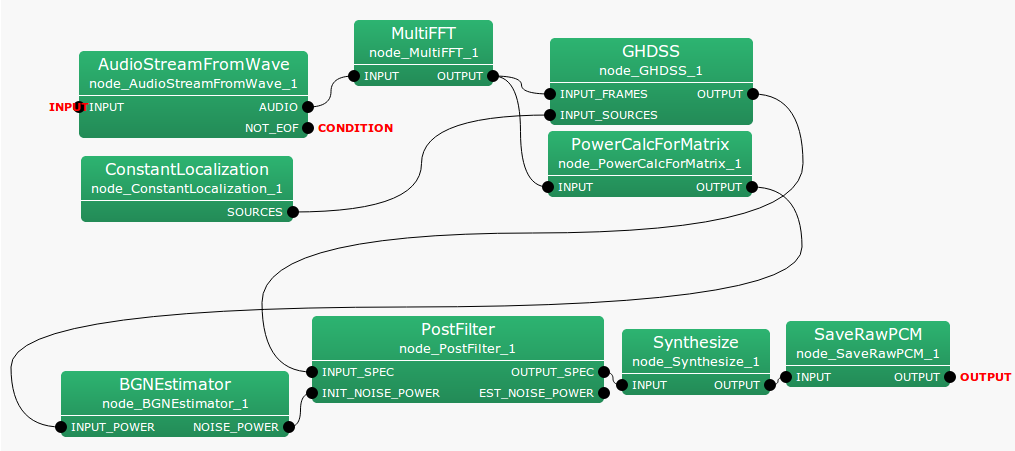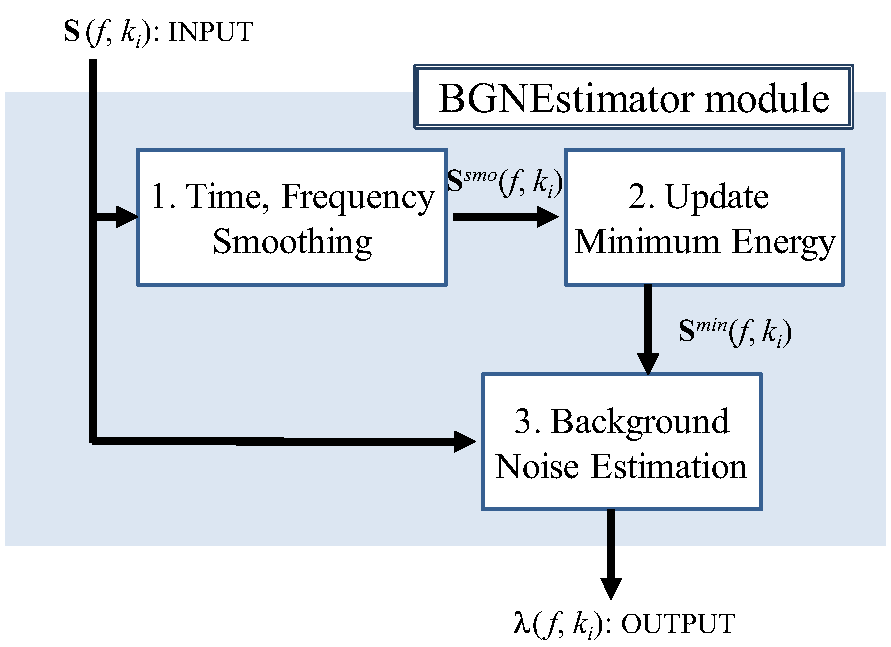6.3.1 BGNEstimator

6.3.1.1 Outline of the node

This node estimates stationary noise (or BackGround Noise) such as fan noise contained in signals, based on the power spectra of multichannel signals. The estimated stationary noise is used in the PostFilter node.

6.3.1.2 Necessary files

No files are required.

6.3.1.3 Usage

When to use

This node is used to estimate stationary noise (or Back Ground Noise) such as fan noise contained in multichannel signals using their power spectra. The node that needs this estimated value is PostFilter . The PostFilter node suppresses the noise that cannot be subtracted by separation processing, based on this background noise and inter-channel leaks estimated in PostFilter . PostFilter estimates stationary noise, though an initial value is needed separately. BGNEstimator is used to generate such an initial value.

Typical connection example

A connection example of the BGNEstimator node is shown in Figure 6.56. As input, the user enters the power spectra that are obtained by converting speech waveforms into the frequency domain. The outputs are used in the PostFilter node.Figure 6.56: Connection example of BGNEstimator

6.3.1.4 Input-output and property of the node

Table 6.40: Parameter list of BGNEstimator
 Parameter name Type Default value Unit Description DELTA 3.0 Power-ratio threshold value L 150 [frame] Detection time width ALPHA_S 0.7 Smoothing coefficient of input signal NOISE_COMPENS 1.0 Mix rate of stationary noise ALPHA_D_MIN 0.05 The minimum value of smoothing coefficient NUM_INIT_FRAME 100 [frame] Number of initialization frames

Input

INPUT_POWER

: Matrix<float> type. Multichannel power spectrum

Output

NOISE_POWER

: Matrix<float> type. Power spectrum of estimated stationary noise.

Parameter

DELTA

: float type. The default value is 3.0. This is the threshold value for determining if the target sound such as speech is included in the frequency bin of a power spectrum. Therefore, the greater the value is, the more quantity of power is judged as stationary noise.

L

: int type. The default value is 150. This is the amount of time to hold the minimum spectrum in history (stationary noise component), which is the criterion for determining the target sound. This parameter is designated in the AudioStreamFromWave node as the number of shifts for the parameter ADVANCE.

ALPHA_S

: float type. The default value is 0.7. The coefficient when smoothing input signals in a temporal direction. The greater the value, the greater we weight the past frame value during smoothing.

NOISE_COMPENS

: float type. The default value is 1.0. This parameter is the weight that weights and adds as stationary noise the frame that is judged not to contain the target sound(smoothing of stationary noise).

ALPHA_D_MIN

: float type. The default value is 0.05. This parameter is the minimum weight when adding the power spectrum of the frame that is judged not to contain the target sound, in the smoothing processing of stationary noise.

NUM_INIT_FRAME

: int type. The default value is 100. When starting the processing, all are judged as stationary noise for the number of frames without judging the presence of the target sound.

6.3.1.5 Details of the node

The process to derive stationary noise is as follows. Time, frequency and channel indices are based on Table 6.1.

Table 6.41: Variable
 Variable name Corresponding parameter or description $\boldsymbol {S}(f,k_ i)= [S_1(f,k_ i), ..., S_ M(f,k_ i)]^ T$ Time frame $f$, input power spectrum of the frequency bin $k_ i$ $\boldsymbol {\lambda }(f,k_ i)= [\lambda _1(f,k_ i), ..., \lambda _ M(f,k_ i)]^ T$ Estimated noise spectrum $\delta$ DELTA, Default value 0.3 $L$ L, default value 150 $\alpha _{s}$ ALPHA_S,Default 0.7 $\theta$ NOISE_COMPENS, Default value 1.0 $\alpha _{d}^{min}$ ALPHA_D_MIN, Default value 0.05 $N$ NUM_INIT_FRAME, default value 100

The derivation flow is as shown in Figure 6.57.Figure 6.57: Flow of stationary noise estimation

1. Time direction, Frequency direction smoothing: Smoothing of the temporal direction is performed by interior division of the input power spectrum ${\boldsymbol S}(f,k_ i)$ and stationary noise power spectrum of the former frame ${\boldsymbol \lambda }(f-1,k_ i)$.

 $\displaystyle S_{m}^{smo,t}(f,k_ i)$ $\displaystyle =$ $\displaystyle \alpha _{s} \lambda _ m(f-1,k_ i)+ (1-\alpha _{s}) S_ m(f,k_ i)$ (34)

Smoothing of the frequency direction is performed for the temporary smoothed time $S_{m}^{smo,t}(f,k_ i)$.

 $\displaystyle S_{m}^{smo}(f,k_ i)$ $\displaystyle =$ $\displaystyle 0.25 S_{m}^{smo}(f,k_{i-1})+ 0.5 S_{m}^{smo}(f,k_ i)+ 0.25 S_{m}^{smo}(f,k_{i+1})$ (35)

2。・Update of the minimum energy: In order to judge presence of the target sound, the minimum energy ${\boldsymbol S}^{min}$ is calculated for each channel and frequency bin after processing is started. ${\boldsymbol S}^{min}$ is the minimum energy for each channel and frequency bin after processing is started ${\boldsymbol S}^{tmp}$ is the provisional minimum energy updated for every $L$ frame.

 $\displaystyle S^{tmp}_ m(f,k_ i)$ $\displaystyle =$ $\displaystyle \left\{ \begin{array}{cr} S^{smo}_ m(f,k_ i), & \mathrm{if}\ \ f = nL\\ \min \{ S^{tmp}_ m(f-1,k_ i), S^{smo}_ m(f,k_ i), & \mathrm{if}\ \ f \neq nL \end{array} \right.$ (36) $\displaystyle S^{min}_ m(f,k_ i)$ $\displaystyle =$ $\displaystyle \left\{ \begin{array}{cr} \min \{ S^{tmp}_ m(f-1,k_ i), S^{smo}_ m(f,k_ i), & {if~ ~ } f = nL\\ \min \{ S^{min}_ m(f-1,k_ i), S^{smo}_ m(f,k_ i), & {if~ ~ } f \neq nL \end{array} \right.$ (37)

Here, $n$ is an arbitrary integer.

3。・Stationary noise estimation:

1. Judgment of the presence of the target sound
When either of the equations below is satisfied, it is judged that power of the target sound is not contained in the concerned time and frequency and only noise exists.

 $\displaystyle S^{smo}_ m(f,k_ i)$ $\displaystyle <$ $\displaystyle \delta S^{min}_ m(f,k_ i) {or}$ (38) $\displaystyle f$ $\displaystyle <$ $\displaystyle N~ {or}$ (39) $\displaystyle S_{m}^{smo}(f,k_ i)$ $\displaystyle <$ $\displaystyle \lambda _ m(f-1,k_ i)$ (40)
2. Calculation of smoothing coefficient
The smoothing coefficient $\alpha _{d}$ used when power of stationary noise is calculated as follows.

 $\displaystyle \alpha _{d}$ $\displaystyle =$ $\displaystyle \left\{ \begin{array}{cr} \frac{1}{f+1}, & {if~ ~ }(\frac{1}{f+1} \geq \alpha _{d}^{min})\\ \alpha _{d}^{min}, & {if~ ~ }(\frac{1}{t+1} < \alpha _{d}^{min})\\ 0 & ({When the target sound is contained}) \end{array} \right.$ (41)

Steady noise is obtained by the following equations.

 $\displaystyle \lambda _ m(f,k_ i)$ $\displaystyle =$ $\displaystyle (1-\alpha _{d}) \lambda _ m(f-1,k_ i)+ \alpha _{d} \theta S_ m(f,k_ i)$ (42)